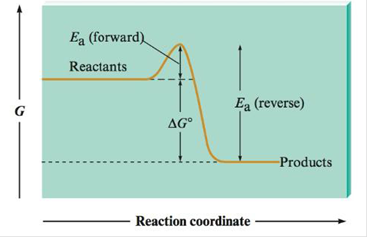# a. Using the free energy profile for a simple one-step reaction, show that at equilibrium K = k f /k r , where k f and k r , are the rate constants for the forward and reverse reactions. Hint: Use the relationship ∆G° = − RT ln( K ) and represent k f and k r , using the Arrhenius equation. ( K = A e − E a / R T ) b. Why is the following statement false? “A catalyst can increase the rate of a forward reaction but not the rote of the reverse reaction.”### Chemistry: An Atoms First Approach

2nd Edition
Steven S. Zumdahl + 1 other
Publisher: Cengage Learning
ISBN: 9781305079243

#### Solutions

Chapter
Section### Chemistry: An Atoms First Approach

2nd Edition
Steven S. Zumdahl + 1 other
Publisher: Cengage Learning
ISBN: 9781305079243
Chapter 16, Problem 109CP
Textbook Problem
5 views

## a. Using the free energy profile for a simple one-step reaction, show that at equilibrium K = kf/kr, where kf and kr, are the rate constants for the forward and reverse reactions. Hint: Use the relationship ∆G° = −RT ln(K) and represent kf and kr, using the Arrhenius equation. ( K = A e − E a / R T )b. Why is the following statement false? “A catalyst can increase the rate of a forward reaction but not the rote of the reverse reaction.”

(a)

Interpretation Introduction

Interpretation: It is to be shown that, K=kfkr by using the free energy profile for a simple one-step solution. The reason of falsity in the statement “A catalyst can increase the rate of a forward reaction, but not the rate of the reverse reaction” is to be stated.

Concept introduction:

To show: At equilibrium, K=kfkr by using the free energy profile for a simple one-step solution.

### Explanation of Solution

The expression for Arrhenius equation is,

k=AeEaRT

Where,

• k is the rate constant.
• A is the Arrhenius parameter.
• Ea is the activation energy.
• R is the universal gas constant.
• T is the absolute temperature.

The rate constants are expressed using the Arrhenius equation,

kf=AeEaRTkr=Ae(EaΔG°)RT

Where,

• kf is the rate constant of forward reaction.
• kr is the rate constant of forward reaction.

The overall rate constant of a reaction is,

k=kfkr (1)

Substitute the values of kf and kr in the above equation

(b)

Interpretation Introduction

Interpretation: It is to be shown that, K=kfkr by using the free energy profile for a simple one-step solution. The reason of falsity in the statement “A catalyst can increase the rate of a forward reaction, but not the rate of the reverse reaction” is to be stated.

Concept introduction:

To show: At equilibrium, K=kfkr by using the free energy profile for a simple one-step solution.

### Still sussing out bartleby?

Check out a sample textbook solution.

See a sample solution

#### The Solution to Your Study Problems

Bartleby provides explanations to thousands of textbook problems written by our experts, many with advanced degrees!

Get Started

Find more solutions based on key concepts
What are three density-independent factors?

Biology (MindTap Course List)

Brown adipose tissue. develops during starvation is a well-known heat-generating tissue develops as fat cells d...

Nutrition: Concepts and Controversies - Standalone book (MindTap Course List)

1-24 Solve: (9.52104)(2.77105)(1.39107)(5.83102)

Introduction to General, Organic and Biochemistry

How can errors in the cell cycle lead to cancer in humans?

Human Heredity: Principles and Issues (MindTap Course List)

What purpose do the colors in a representational-color image or map serve?

Horizons: Exploring the Universe (MindTap Course List)

What is a pheromone?

Organic And Biological Chemistry

Some __________ can cause cancer in humans. a. viruses b. bacteria c. viroids d. a and b

Biology: The Unity and Diversity of Life (MindTap Course List)

Give examples of marine flowering plants. Are they vascular plants or nonvascular algae?

Oceanography: An Invitation To Marine Science, Loose-leaf Versin

Review. At what temperature would the average speed of helium atoms equal (a) the escape speed from the Earth, ...

Physics for Scientists and Engineers, Technology Update (No access codes included)